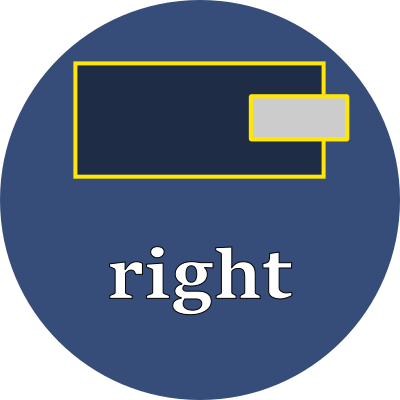# rightExplicitly set the right edge of the element.

## Property values

This property has different interpretations, depending upon the element's `position` property.

position interpretation
static This property is ignored.
relative Shifts the normal flow-of-text position by the given amount. Positive values shift it inside the element's right boundary. Negative values shift it outside the element's right boundary.
fixed Sets the right edge of the element to be the given amount inside the right edge of the browser's viewport.
absolute Sets the right edge of the element to be the given amount inside the right edge of the ancestor's viewport, but only if that ancestor has a position that is `relative`, `fixed` or `absolute`.
sticky Follows the rules for `relative` until the right value is scrolled to. After that, follows the rules for `fixed` and uses the given value as a fixed amount from the browser's right edge.

### Units

Use any of these font-relative typographic units to set the right:

 em em The `font-size` of the current font rem relative em The `font-size` of the element ch "0" The advance measure (the width) of the current font's "0" glyph ic ideograph count The advance measure (the width) of the current font's "水" ideograph

Or use any of these fixed size units which are independent of the current font:

 % percent A percentage of the parent element's width in inch A size equal 96px px pixels A size equal to 1/96 inch pt point A size equal 1in/72 = 1.33px pc pica A size equal 1in/6 = 12pt = 16px cm centimeter A size equal to 96px/2.54 = 37.8px mm millimeter A size equal to 96px/25.4 = 3.8px Q quarter millimeter A size equal to 96px/1016 = 0.94px

## Examples

style > position > rightRight edge of element